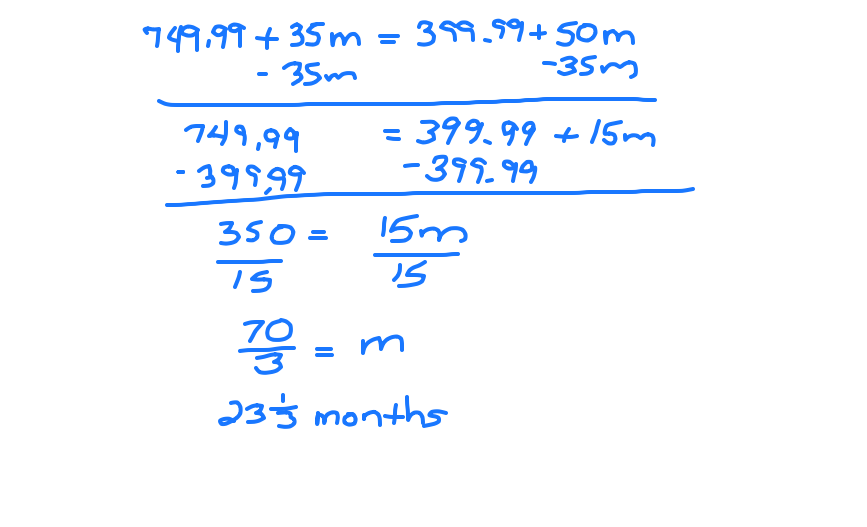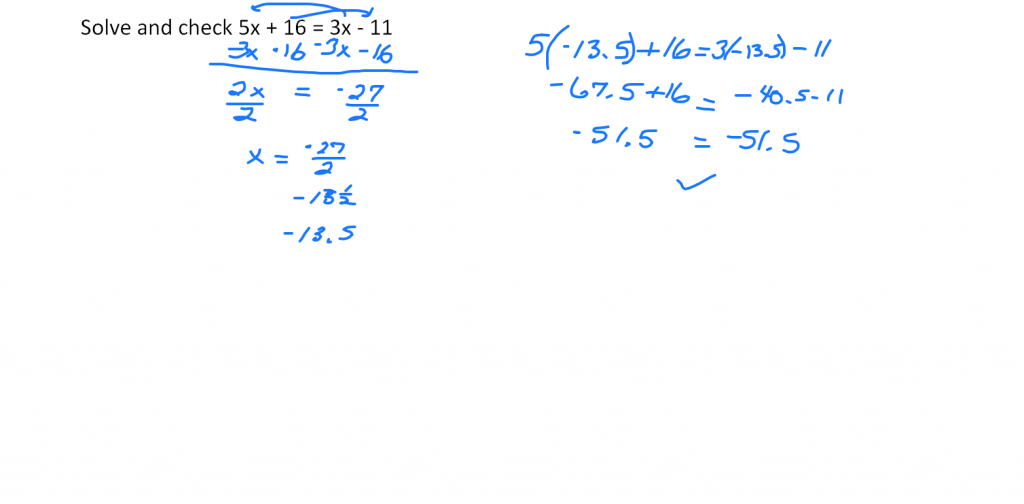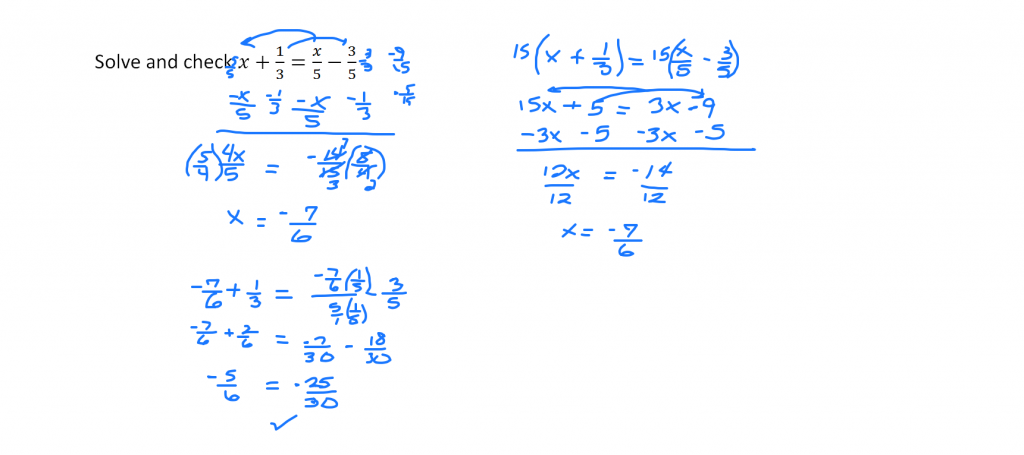# Multi-Step Equations

//Multi-Step Equations
Multi-Step Equations 2017-11-13T22:01:52+00:00Boost Mobile™ offers a 64GB iPhone® 6 Plus for \$749.99 and unlimited talk, text, and 1 GB of high speed data for \$35/mo. with no contract.

AT&T™ offers a 64GB iPhone® 6 Plus for \$399.99 and unlimited talk, text and a 1 GB high speed data plan for \$50/mo. but requires a two year contract.

How many months will it take before the two options cost the same amount?

Write an expression for both plans and set them equal to one another.

749.99 + 35m = 399.99 + 50m

Solve by bringing the variables to one side of the equation and the constants to the other side. Then divide both sides by the number multiplied by the variable.Solve 5x + 16 = 3x – 11 and check the result.

Begin by bringing the variables to one side. Subtract 3x from both sides. If you have had enough practice on these problems and won’t get confused, bring the constants to the other side and subtract 16 from both sides in the same steps. The result is 2x = -27. Since 2 is taken times x, divide both sides by 2. The result is -13.5.Verify your results by checking. Plug -13.5 into each instance of x.

For the final example, you can work the problem two ways. You can get a common denominator with the fractions, or you can multiply both sides by the LCM. Both ways are shown.Holt, Rinehart and Winston Algebra 1 p. 135 – 140